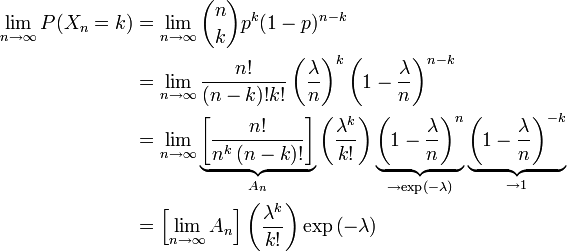# Closing In. Perhaps.

This website is great.

Now I learn that the best way of thinking of exponents is to think of them as causing numbers to grow. See the link.

And e is the ‘natural’ rate of growth for all things in the universe. Strange metaphor, calling it ‘natural’.

I’m now starting to think of it as the smooth growth version of 1 + 1. Putting two 1s together is the simplest arithmetic. The exponential version of this operation gives you 2.71, not 2. This quote provides an interesting clue:

Every exponent is a variation of e, just like every number is a scaled version of 1.

So if every number in the world is just a bunch of 1s added together (the 1 + 1 operation preformed lots of times), then every exponential operation is just some variation on e.

So e doesn’t need to be a mystery, it’s the default growth rate, like 1 is the default number.

Back to Poisson, where this is giving me another clue:That’s a bunch of incomprehensible math-blag, but the point here is that the [] term = 1, so the Poisson is just a nifty short-hand way of doing the binomial distribution with lots of n. And the binomial distribution is just picking things with some probability of success.

Now remember lambda is our expected number of ‘hits’ and so 1/lamba is the probability of any hit. All the exp(-lambda) (ie. exp[1/lambda]) does is grow us a cumulative probability threshold, which we can hit with a series of p = 0.5 random events.

I’m getting closer?

## One thought on “Closing In. Perhaps.”

This site uses Akismet to reduce spam. Learn how your comment data is processed.